Julia Fractals in JavaScript

Originally written for Hack Club, a global network of high-school hackers and coding clubs.

Fractals. You've seen them. They're beautiful:What kind of crazy black magic lets computers draw these things?? It must be like… really complicated.

WRONG. Fractal renderers are ridiculously simple—and to prove it, you're going to write one.

``````<!DOCTYPE html>
<html>
<title>Julia Fractals</title>

<style>
body {
background: black;
color: white;
}
canvas {
border: 1px solid white;
}
</style>

<body>
<h2></h2>
<canvas></canvas>
</body>

<!-- Import math.js -->
<script src="https://cdnjs.cloudflare.com/ajax/libs/mathjs/5.6.0/math.min.js"></script>

<script>

// The HTML elements we are using
var canvas = document.querySelector('canvas')
var ctx = canvas.getContext('2d')

// The size of our canvas
var width = 200
var height = 200

// Set the size of our canvas
canvas.width = width
canvas.height = height

// The XY coordinates of the mouse over the canvas
var mouseX = 0
var mouseY = 0

</script>
</html>``````

## The Complex Plane

Fractals arise from basic arithmetic with complex numbers. Despite their name, complex numbers are actually quite simple; instead of a number line, complex numbers exist on a plane.

Complex numbers are usually written `x+y*i`. So `2+4i` is at the point (2, 4).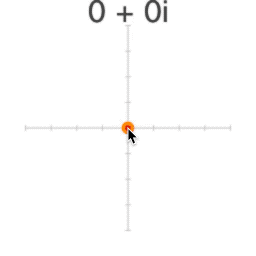`i` is the square root of -1 (so `i*i=-1`). We can't find a value for `i` on the "real" number line, because `x*x` is never negative.

For a long time, mathematicians just ignored square roots of negative numbers—just like they used to ignore negative numbers. They aren't "real" numbers, so what good are they?

Then somebody thought to put `i` on its own separate, vertical number line. The Complex Plane was born—you won't believe what happened next.

### From Pixels to Points

Each pixel on our canvas corresponds to a point on the complex plane. We need a function to convert XY pixels to complex points.

Complex numbers aren't "built in" for most programming languages. We'll use a library called math.js to work with them in JavaScript. To create a new complex number with math.js, we simply write `math.complex(x, y)`

Add a function called `pixelToPoint` to the end of your script:

``````// the rest of your script...

// Turn XY pixel coordinates into a point on the complex plane
function pixelToPoint(x, y) {
// Map percentage of total width/height to a value from -1 to +1
var zx = (x/width)*2-1
var zy = 1-(y/height)*2

// Create a complex number based on our new XY values
return math.complex(zx, zy)
}``````

This takes the X and Y coordinates for a pixel, divides each to get that pixel's percentage of the overall width/height, and maps that percentage to a value from -1 to +1. The result is a point between `-1-1i` and `1+1i`.

Note how `zx` is `(x/width)*2-1`, but `zy` is `1-(y/height)*2`. `zy` is flipped—this is just because on a webpage, pixel coordinates go top-to-bottom (and we want our complex plane to be bottom-to-top).

Now let's use that function to pick out a point on the plane with the mouse. Later on we'll need a point called `constant` for our fractal equation, so let's use that.

Add a complex number called `constant` to the top of your script, just before `pixelToPoint` and just after `mouseX`/`mouseY`:

``````// mouseX/mouseY + the rest of your script...

// The point we use for C in our Julia Set equation
var constant = math.complex(0.28, 0.01)

// pixelToPoint + the rest of your script...``````

Now let's hook up the mouse to the canvas.

Add two new functions called `update` and `move` to the end of your script. `move` will fire every time the mouse moves to a new point on the canvas, and `update` will change the header to show that point.

We'll also add an event listener to the canvas to trigger `move`:

``````// pixelToPoint + the rest of your script...

// Update the elements that need to change
function update() {
}

// What to do when the mouse moves over the canvas
function move(event) {
// Get the mouse's XY coordinates on canvas
mouseX = event.clientX-canvas.offsetLeft
mouseY = event.clientY-canvas.offsetTop

// Turn mouse coordinates into a point on the complex plane
constant = pixelToPoint(mouseX, mouseY)

// Round that point off to the nearest 0.01
constant.re = math.round(constant.re*100)/100
constant.im = math.round(constant.im*100)/100

// Update everything!
update()
}

// Trigger move every time the mouse moves over canvas

Note that we're rounding `constant` to the nearest 0.01:

``````constant.re = math.round(constant.re*100)/100
constant.im = math.round(constant.im*100)/100``````

This just looks a little neater. `constant.re` is the "real" part of the number—the X value. `constant.im` is the "imaginary" part—the Y value.

Now if you run the page and mouse over the canvas, you should see `constant` updating in the header!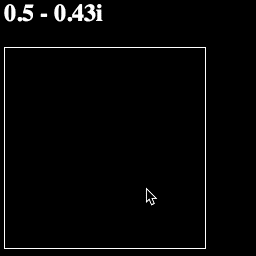Your whole script should look like this:

``````<script>

// The HTML elements we are using
var canvas = document.querySelector('canvas')
var ctx = canvas.getContext('2d')

// The size of our canvas
var width = 200
var height = 200

// Set the size of our canvas
canvas.width = width
canvas.height = height

// The XY coordinates of the mouse over the canvas
var mouseX = 0
var mouseY = 0

// The point we use for C in our Julia Set equation
var constant = math.complex(0.28, 0.01)

// Turn XY pixel coordinates into a point on the complex plane
function pixelToPoint(x, y) {
// Map percentage of total width/height to a value from -1 to +1
var zx = (x/width)*2-1
var zy = 1-(y/height)*2

// Create a complex number based on our new XY values
return math.complex(zx, zy)
}

// Update the elements that need to change
function update() {
}

// What to do when the mouse moves over the canvas
function move(event) {
// Get the mouse's XY coordinates on canvas
mouseX = event.clientX-canvas.offsetLeft
mouseY = event.clientY-canvas.offsetTop

// Turn mouse coordinates into a point on the complex plane
constant = pixelToPoint(mouseX, mouseY)

// Round that point off to the nearest 0.01
constant.re = math.round(constant.re*100)/100
constant.im = math.round(constant.im*100)/100

// Update everything!
update()
}

// Trigger move every time the mouse moves over canvas

</script>``````

## Drawing Pixels

Let's draw some colors on our canvas. Every pixel maps to a point, and every point maps to a color.

Let's make it so that every time we change `constant` we draw in a color for that pixel.

To do this, we need a new function called `pointToColor`. This function will turn any point into an RGB color, simply by using the "real" (X) value `point.re` for Red and the "imaginary" (Y) value `point.im` for green:

``````// constant + the rest of your script...

// Turn a point on the complex plane into a color
function pointToColor(point) {
var red = point.re*255
var green = point.im*255
return `rgb(\${red}, \${green}, 0)`
}

// pixelToPoint + the rest of your script...``````

We will also need two more functions, called `drawPixel` and `draw`.

`drawPixel` will draw a given color at a given pixel. `draw` will

``````// pixelToPoint + the rest of your script...

// Draw a single pixel on our canvas
function drawPixel(x, y, color) {
ctx.fillStyle = color
ctx.fillRect(x, y, 1, 1)
}

// Redraw our canvas
function draw() {
// Turn the point under the mouse into a color
var color = pointToColor(constant)

// Draw over the pixel under the mouse with that color
drawPixel(mouseX, mouseY, color)
}

// update + the rest of your script...``````

Let's quickly look at `drawPixel`. This is how basic drawing works with canvas.

First you set properties like `fillStyle` on the "drawing context" (usually labelled `ctx`). Then you trigger a drawing operation like `fillRect` to draw shapes onto the canvas—in this case, a single 1x1 rectangle.

Finally, we need to add `draw()` to `update` so that our canvas will redraw anytime we call `update`:

``````// draw + the rest of your script...

// Update the elements that need to change
function update() {
draw()
}

// move + the rest of your script...``````

Now if you run the page, you should see little colored pixels show up as you mouse over the plane: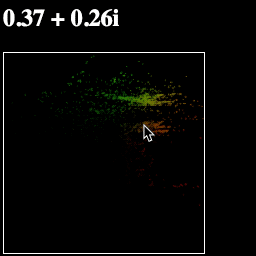Your script should now look like this:

``````<script>

// The HTML elements we are using
var canvas = document.querySelector('canvas')
var ctx = canvas.getContext('2d')

// The size of our canvas
var width = 200
var height = 200

// Set the size of our canvas
canvas.width = width
canvas.height = height

// The XY coordinates of the mouse over the canvas
var mouseX = 0
var mouseY = 0

// The point we use for C in our Julia Set equation
var constant = math.complex(0.28, 0.01)

// Turn a point on the complex plane into a color
function pointToColor(point) {
var red = point.re*255
var green = point.im*255
return `rgb(\${red}, \${green}, 0)`
}

// Turn XY pixel coordinates into a point on the complex plane
function pixelToPoint(x, y) {
// Map percentage of total width/height to a value from -1 to +1
var zx = (x/width)*2-1
var zy = 1-(y/height)*2

// Create a complex number based on our new XY values
return math.complex(zx, zy)
}

// Draw a single pixel on our canvas
function drawPixel(x, y, color) {
ctx.fillStyle = color
ctx.fillRect(x, y, 1, 1)
}

// Redraw our canvas
function draw() {
// Turn the point under the mouse into a color
var color = pointToColor(constant)

// Draw over the pixel under the mouse with that color
drawPixel(mouseX, mouseY, color)
}

// Update the elements that need to change
function update() {
draw()
}

// What to do when the mouse moves over the canvas
function move(event) {
// Get the mouse's XY coordinates on canvas
mouseX = event.clientX-canvas.offsetLeft
mouseY = event.clientY-canvas.offsetTop

// Turn mouse coordinates into a point on the complex plane
constant = pixelToPoint(mouseX, mouseY)

// Round that point off to the nearest 0.01
constant.re = math.round(constant.re*100)/100
constant.im = math.round(constant.im*100)/100

// Update everything!
update()
}

// Trigger move every time the mouse moves over canvas

</script>``````

## Drawing the Plane

We don't just want to draw one pixel at a time—we want the whole plane at once.

Let's modify our `draw` function so it fills in every pixel:

``````// drawPixel + the rest of your script

// Redraw our canvas
function draw() {
// Loop over every column of pixels
for (var y = 0; y < height; y++) {
// Loop over every row of pixels
for (var x = 0; x < width; x++) {
// Turn this pixel into a point in the complex plane
var point = pixelToPoint(x, y)

// Turn that point into a color
var color = pointToColor(point)

// Draw over this pixel with that color
drawPixel(x, y, color)
}
}
}

// update + the rest of your script...``````

This new `draw` function is actually pretty simple. It uses two loops—one for X and one for Y—to go over every pixel in our canvas. Then it gets the complex plane point for that pixel. Then it gets the color for that point. Then it draws that color at that pixel.

If you run the page, you should see the same field of red/green pixels—only now they are all filled in!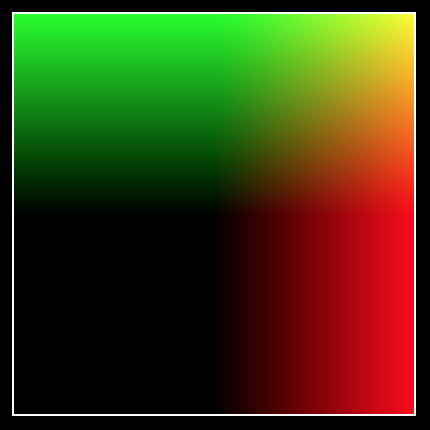The only problem is that the page waits for the mouse to move before drawing the canvas. Let's fix that with a call to `update` at the end of our script, just after we add the event listener:

``````// addEventListener + the rest of your script...

// Update everything!
update()``````

### Simple Complex Arithmetic

Let's make a few more changes to our `pointToColor` function, just to understand what's going on. First, try adding

`point = point.sub(constant)`

To the top of `pointToColor`:

``````// constant + the rest of your script...

// Turn a point on the complex plane into a color
function pointToColor(point) {
point = point.sub(constant)

var red = point.re*255
var green = point.im*255

return `rgb(\${red}, \${green}, 0)`
}

// pixelToPoint + the rest of your script...``````

This means we subtract `constant` from our `point` before we turn it into a color. Since complex numbers aren't built into JavaScript, we can't use `+-*/`—math.js has us use `.add()` `.sub()` `.mul()` `.div()` instead.

What does it mean to add/subtract a complex number? Well, it's pretty much the same as adding regular numbers: 1+2i + 1+2i = 2+4i.

However, there is a better, more geometric way to think about this: we are using one point to move another point around the plane: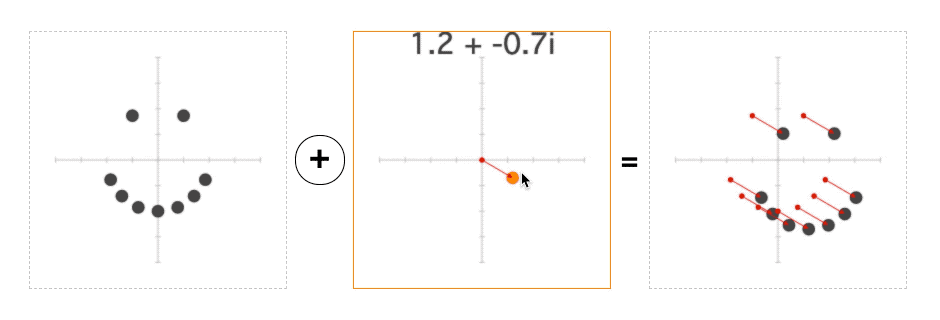And if you run the page, this is exactly what you should see—when we subtract the mouse position from each point before turning it into a color, the entire plane will move with the mouse: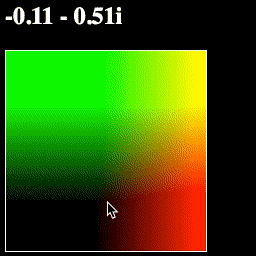What a convenient geometric representation!

As it turns out, you can multiply complex numbers too. Amazingly, this also has a simple geometric interpretation: rotation and scaling: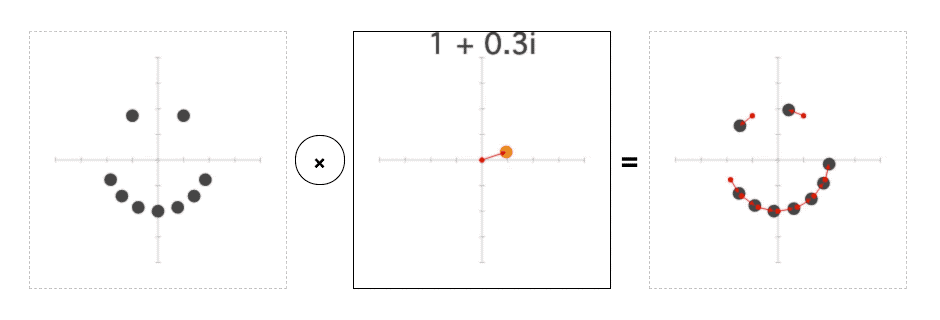Try changing this line:

`point = point.sub(constant)`

to this:

`point = point.div(constant)`

This will divide each point on the plane by `constant` before turning it into a color. And if you run the page, you should see the plane rotate and scale with the mouse: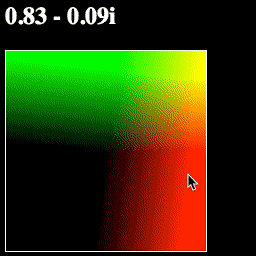This way of thinking about complex numbers—where add/subtract moves a point, and multiply/divide rotates and scales it—is incredibly powerful.

Let's make one more change, to show off an important function called `math.abs`. The Absolute Value of a complex number is its "length"—the distance from that point on the complex plane to 0+0i.

Try changing your `pointToColor` function like so:

``````// constant + the rest of your script...

// Turn a point on the complex plane into a color
function pointToColor(point) {
point = point.div(constant)

var red = point.re*255
var green = point.im*255
var blue = math.abs(point)*255

return `rgb(\${red}, \${green}, \${blue})`
}

// pixelToPoint + the rest of your script...``````

Now we have a `blue` value for our color, which shows `math.abs(point)`. If you run the page, it should look like this: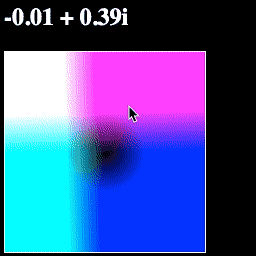Think about the colors you see—why these colors? Why is the blue in a circle? Why is one corner white?

Your script should now look like this:

``````<script>

// The HTML elements we are using
var canvas = document.querySelector('canvas')
var ctx = canvas.getContext('2d')

// The size of our canvas
var width = 200
var height = 200

// Set the size of our canvas
canvas.width = width
canvas.height = height

// The XY coordinates of the mouse over the canvas
var mouseX = 0
var mouseY = 0

// The point we use for C in our Julia Set equation
var constant = math.complex(0.28, 0.01)

// Turn a point on the complex plane into a color
function pointToColor(point) {
point = point.sub(constant)

var red = point.re*255
var green = point.im*255
var blue = math.abs(point)*255

return `rgb(\${red}, \${green}, \${blue})`
}

// Turn XY pixel coordinates into a point on the complex plane
function pixelToPoint(x, y) {
// Map percentage of total width/height to a value from -1 to +1
var zx = (x/width)*2-1
var zy = 1-(y/height)*2

// Create a complex number based on our new XY values
return math.complex(zx, zy)
}

// Draw a single pixel on our canvas
function drawPixel(x, y, color) {
ctx.fillStyle = color
ctx.fillRect(x, y, 1, 1)
}

// Redraw our canvas
function draw() {
// Loop over every column of pixels
for (var y = 0; y < height; y++) {
// Loop over every row of pixels
for (var x = 0; x < width; x++) {
// Turn this pixel into a point in the complex plane
var point = pixelToPoint(x, y)

// Turn that point into a color
var color = pointToColor(point)

// Draw over this pixel with that color
drawPixel(x, y, color)
}
}
}

// Update the elements that need to change
function update() {
draw()
}

// What to do when the mouse moves over the canvas
function move(event) {
// Get the mouse's XY coordinates on canvas
mouseX = event.clientX-canvas.offsetLeft
mouseY = event.clientY-canvas.offsetTop

// Turn mouse coordinates into a point on the complex plane
constant = pixelToPoint(mouseX, mouseY)

// Round that point off to the nearest 0.01
constant.re = math.round(constant.re*100)/100
constant.im = math.round(constant.im*100)/100

// Update everything!
update()
}

// Trigger move every time the mouse moves over canvas

// Update everything!
update()

</script>``````

## The Julia Set

Now for the main event—rendering a fractal.

We're going to render the Julia Set. This fractal is defined by a very simple function: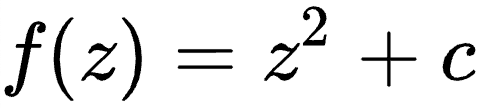We need to use this equation to generate a color for each point on the complex plane. However, this function doesn't give you a color directly—it defines a process that you can apply to each point:

1. Multiply that point by itself
2. Add your `constant` value C
3. Repeat
1. Multiply this new point by itself
2. Add your `constant` value C
3. Repeat
1. Multiply this new point by itself
2. Add your `constant` value C
3. Repeat…

You can apply this process infinitely for any point. For most points, the number will just keep getting bigger and bigger and bigger. When this happens, we say the number Escapes.

Any point with an absolute value of at least 2 will always get bigger and bigger forever. So if `math.abs(z) >= 2`, we know that `z` escapes.

But for some numbers in that little circle around the origin—where `math.abs(z) < 2`—something peculiar happens. Each time we apply this process, the point will move… but it will never escape. It may just bounce around forever, rotating and moving around the origin without ever "escaping" it.

To get a color from each point, we apply this process a bunch of times to see if that point escapes. We stop when `math.abs(z) > 2`, or when we hit some maximum number of iterations (we'll set a max of 64).

Add a new value called `maxIterations` just below `constant`, and a new function called `julia` just after that:

``````// constant + the rest of your script...

// The maximum number of times we iterate a point to see if it escapes
var maxIterations = 64

// Apply the Julia Set formula to see if point z "escapes"
function julia(z, i = 0) {
// Apply the Julia Set formula: z*z+constant
z = z.mul(z)

// Has our point escaped, or hit the iteration limit?
if (math.abs(z) > 2 || i == maxIterations)
// If so, return number of iterations
return i
else
// If not, iterate again!
return julia(z, i+1)
}

// pointToColor + the rest of your script...``````

Now we have a function `julia` which tells us how many iterations of the Julia Set equation it takes for a given point to escape.

We need to turn that number of iterations into a color. We'll use a simple grayscale color scheme, where black is 0 iterations and white is our `maxIterations` value.

Change your `pointToColor` function like so:

``````// julia + the rest of your script...

// Turn a point on the complex plane into a color
function pointToColor(point) {
// How many iterations on this point before it escapes?
var iterations = julia(point)

// What percentage of our limit is that?
var percentage = iterations/maxIterations

var red = percentage*255
var green = percentage*255
var blue = percentage*255

// Create a color from that percentage
return `rgb(\${red}, \${green}, \${blue})`
}

// pixelToPoint + the rest of your script...``````

Now if your run your page, you should see a grayscale Julia fractal that morphs as your move the mouse: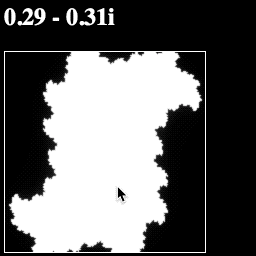Your whole script should now look like this:

``````<script>

// The HTML elements we are using
var canvas = document.querySelector('canvas')
var ctx = canvas.getContext('2d')

// The size of our canvas
var width = 200
var height = 200

// Set the size of our canvas
canvas.width = width
canvas.height = height

// The XY coordinates of the mouse over the canvas
var mouseX = 0
var mouseY = 0

// The point we use for C in our Julia Set equation
var constant = math.complex(0.28, 0.01)

// The maximum number of times we iterate a point to see if it escapes
var maxIterations = 64

// Apply the Julia Set formula to see if point z "escapes"
function julia(z, i = 0) {
// Apply the Julia Set formula: z*z+constant
z = z.mul(z)

// Has our point escaped, or hit the iteration limit?
if (math.abs(z) > 2 || i == maxIterations)
// If so, return number of iterations
return i
else
// If not, iterate again!
return julia(z, i+1)
}

// Turn a point on the complex plane into a color
function pointToColor(point) {
// How many iterations on this point before it escapes?
var iterations = julia(point)

// What percentage of our limit is that?
var percentage = iterations/maxIterations

var red = percentage*255
var green = percentage*255
var blue = percentage*255

// Create a color from that percentage
return `rgb(\${red}, \${green}, \${blue})`
}

// Turn XY pixel coordinates into a point on the complex plane
function pixelToPoint(x, y) {
// Map percentage of total width/height to a value from -1 to +1
var zx = (x/width)*2-1
var zy = 1-(y/height)*2

// Create a complex number based on our new XY values
return math.complex(zx, zy)
}

// Draw a single pixel on our canvas
function drawPixel(x, y, color) {
ctx.fillStyle = color
ctx.fillRect(x, y, 1, 1)
}

// Redraw our canvas
function draw() {
// Loop over every column of pixels
for (var y = 0; y < height; y++) {
// Loop over every row of pixels
for (var x = 0; x < width; x++) {
// Turn this pixel into a point in the complex plane
var point = pixelToPoint(x, y)

// Turn that point into a color
var color = pointToColor(point)

// Draw over this pixel with that color
drawPixel(x, y, color)
}
}
}

// Update the elements that need to change
function update() {
draw()
}

// What to do when the mouse moves over the canvas
function move(event) {
// Get the mouse's XY coordinates on canvas
mouseX = event.clientX-canvas.offsetLeft
mouseY = event.clientY-canvas.offsetTop

// Turn mouse coordinates into a point on the complex plane
constant = pixelToPoint(mouseX, mouseY)

// Round that point off to the nearest 0.01
constant.re = math.round(constant.re*100)/100
constant.im = math.round(constant.im*100)/100

// Update everything!
update()
}

// Trigger move every time the mouse moves over canvas

// Update everything!
update()

</script>``````

## Pan

Now we have a fractal, which is pretty cool. But what if we want to move around within our fractal?

Let's start with a "pan" operation, that moves the image up/down/left/right as we click.

We'll set this up so that the first click sets our `constant` value, and every click after that pans the image.

Add two new values for `clicked` and `pan` at the top, just below `maxIterations` and above `julia`:

``````// maxIterations + the rest of your script...

// Whether we have clicked yet
var clicked = false

// How much we move the image
var pan = math.complex(0, 0)

// julia + the rest of your script...``````

Now add a new function called `click`, just after `update`:

``````// update + the rest of your script...

function click(event) {
// Ignore the first click
if (!clicked) {
clicked = true
return
}

// Get the mouse's XY coordinates on canvas
mouseX = event.clientX-canvas.offsetLeft
mouseY = event.clientY-canvas.offsetTop

// Turn mouse coordinates into a point on the complex plane
pan = pixelToPoint(mouseX, mouseY)

// Update everything!
update()
}

// the rest of your script...``````

Notice that if `clicked` is false, `click` will do nothing—except set `clicked` to true.

Similarly, we need to change `move` so that it will do nothing if `clicked` is true:

``````// click + the rest of your script...

// What to do when the mouse moves over the canvas
function move(event) {
// Don't move after first click
if (clicked) {
return
}

// Get the mouse's XY coordinates on canvas
mouseX = event.clientX-canvas.offsetLeft
mouseY = event.clientY-canvas.offsetTop

// Turn mouse coordinates into a point on the complex plane
constant = pixelToPoint(mouseX, mouseY)

// Round that point off to the nearest 0.01
constant.re = math.round(constant.re*100)/100
constant.im = math.round(constant.im*100)/100

// Update everything!
update()
}``````

We also need to add a listener to trigger `click` whenever we click on the canvas. Let's put it just after our `move` function:

``````// move + the rest of your script...

// Trigger click every time the canvas is clicked

// the rest of your script...``````

Now when we click on the canvas, it will fix our `constant` value—and every click after that will change the `pan` value.

Finally, we need to actually use that `pan` value somewhere.

We want `pan` to change which pixel maps to each point—so we need to change our `pixelToPoint` function so that our `pan` value is added to each point:

``````// pointToColor + the rest of your script...

// Turn XY pixel coordinates into a point on the complex plane
function pixelToPoint(x, y) {
// Map percentage of total width/height to a value from -1 to +1
var zx = (x/width)*2-1
var zy = 1-(y/height)*2

// Create a complex number based on our new XY values
var z = math.complex(zx, zy)

// Pan the camera

return z
}

// drawPixel + the rest of your script...``````

Now if you run the page, you should be able to set the `constant` value and pan around the image by clicking!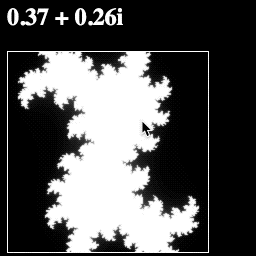Your full script should look like this:

``````<script>

// The HTML elements we are using
var canvas = document.querySelector('canvas')
var ctx = canvas.getContext('2d')

// The size of our canvas
var width = 200
var height = 200

// Set the size of our canvas
canvas.width = width
canvas.height = height

// The XY coordinates of the mouse over the canvas
var mouseX = 0
var mouseY = 0

// The point we use for C in our Julia Set equation
var constant = math.complex(0.28, 0.01)

// The maximum number of times we iterate a point to see if it escapes
var maxIterations = 64

// Whether we have clicked yet
var clicked = false

// How much we move the image
var pan = math.complex(0, 0)

// Apply the Julia Set formula to see if point z "escapes"
function julia(z, i = 0) {
// Apply the Julia Set formula: z*z+constant
z = z.mul(z)

// Has our point escaped, or hit the iteration limit?
if (math.abs(z) > 2 || i == maxIterations)
// If so, return number of iterations
return i
else
// If not, iterate again!
return julia(z, i+1)
}

// Turn a point on the complex plane into a color
function pointToColor(point) {
// How many iterations on this point before it escapes?
var iterations = julia(point)

// What percentage of our limit is that?
var percentage = iterations/maxIterations

var red = percentage*255
var green = percentage*255
var blue = percentage*255

// Create a color from that percentage
return `rgb(\${red}, \${green}, \${blue})`
}

// Turn XY pixel coordinates into a point on the complex plane
function pixelToPoint(x, y) {
// Map percentage of total width/height to a value from -1 to +1
var zx = (x/width)*2-1
var zy = 1-(y/height)*2

// Create a complex number based on our new XY values
var z = math.complex(zx, zy)

// Pan the camera

return z
}

// Draw a single pixel on our canvas
function drawPixel(x, y, color) {
ctx.fillStyle = color
ctx.fillRect(x, y, 1, 1)
}

// Redraw our canvas
function draw() {
// Loop over every column of pixels
for (var y = 0; y < height; y++) {
// Loop over every row of pixels
for (var x = 0; x < width; x++) {
// Turn this pixel into a point in the complex plane
var point = pixelToPoint(x, y)

// Turn that point into a color
var color = pointToColor(point)

// Draw over this pixel with that color
drawPixel(x, y, color)
}
}
}

// Update the elements that need to change
function update() {
draw()
}

function click(event) {
// Ignore the first click
if (!clicked) {
clicked = true
return
}

// Get the mouse's XY coordinates on canvas
mouseX = event.clientX-canvas.offsetLeft
mouseY = event.clientY-canvas.offsetTop

// Turn mouse coordinates into a point on the complex plane
pan = pixelToPoint(mouseX, mouseY)

// Update everything!
update()
}

// What to do when the mouse moves over the canvas
function move(event) {
// Don't move after first click
if (clicked) {
return
}

// Get the mouse's XY coordinates on canvas
mouseX = event.clientX-canvas.offsetLeft
mouseY = event.clientY-canvas.offsetTop

// Turn mouse coordinates into a point on the complex plane
constant = pixelToPoint(mouseX, mouseY)

// Round that point off to the nearest 0.01
constant.re = math.round(constant.re*100)/100
constant.im = math.round(constant.im*100)/100

// Update everything!
update()
}

// Trigger click every time the canvas is clicked

// Trigger move every time the mouse moves over canvas

// Update everything!
update()

</script>``````

## Zoom

Almost done here… all we need now is a zoom feature.

Add a new variable for `zoom`, just after `pan`:

``````// pan + the rest of your script...

// How much we zoom the image
var zoom = 1

// julia + the rest of your script...``````

Let's make it so every click doubles the zoom value.

We just need to add one new line to our `click` function for `zoom *= 2`:

``````// update + the rest of your script...

// What to do when the mouse clicks the canvas
function click(event) {
// Ignore the first click
if (!clicked) {
clicked = true
return
}

// Get the mouse's XY coordinates on canvas
mouseX = event.clientX-canvas.offsetLeft
mouseY = event.clientY-canvas.offsetTop

// Turn mouse coordinates into a point on the complex plane
pan = pixelToPoint(mouseX, mouseY)

// Zoom in twice as far
zoom *= 2

// Update everything!
update()
}

// move + the rest of your script...``````

Now we need to change our `pixelToPoint` function to use that `zoom` value. Like `pan`, this is pretty simple—we just need to divide each point by `zoom`, just before we add `pan`:

``````// pointToColor + the rest of your script...

// Turn XY pixel coordinates into a point on the complex plane
function pixelToPoint(x, y) {
// Map percentage of total width/height to a value from -1 to +1
var zx = (x/width)*2-1
var zy = 1-(y/height)*2

// Create a complex number based on our new XY values
var z = math.complex(zx, zy)

// Zoom the camera
z = z.div(zoom)

// Pan the camera

return z
}

// drawPixel + the rest of your script...``````

Finally, let's make one last change to our `update` function so that it will tell us how far we have zoomed in:

``````// draw + the rest of your script...

// Update the elements that need to change
function update() {
header.innerHTML = constant.toString() + " at " + zoom + "X"
draw()
}

// click + the rest of your script...``````

Now if you run the page, you should be able to zoom in on the image with every click!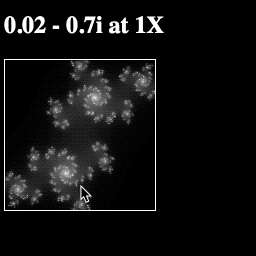Your final script should look like this:

``````<script>

// The HTML elements we are using
var canvas = document.querySelector('canvas')
var ctx = canvas.getContext('2d')

// The size of our canvas
var width = 200
var height = 200

// Set the size of our canvas
canvas.width = width
canvas.height = height

// The XY coordinates of the mouse over the canvas
var mouseX = 0
var mouseY = 0

// The point we use for C in our Julia Set equation
var constant = math.complex(0.28, 0.01)

// The maximum number of times we iterate a point to see if it escapes
var maxIterations = 64

// Whether we have clicked yet
var clicked = false

// How much we move the image
var pan = math.complex(0, 0)

// How much we zoom the image
var zoom = 1

// Apply the Julia Set formula to see if point z "escapes"
function julia(z, i = 0) {
// Apply the Julia Set formula: z*z+constant
z = z.mul(z)

// Has our point escaped, or hit the iteration limit?
if (math.abs(z) > 2 || i == maxIterations)
// If so, return number of iterations
return i
else
// If not, iterate again!
return julia(z, i+1)
}

// Turn a point on the complex plane into a color
function pointToColor(point) {
// How many iterations on this point before it escapes?
var iterations = julia(point)

// What percentage of our limit is that?
var percentage = iterations/maxIterations

var red = percentage*255
var green = percentage*255
var blue = percentage*255

// Create a color from that percentage
return `rgb(\${red}, \${green}, \${blue})`
}

// Turn XY pixel coordinates into a point on the complex plane
function pixelToPoint(x, y) {
// Map percentage of total width/height to a value from -1 to +1
var zx = (x/width)*2-1
var zy = 1-(y/height)*2

// Create a complex number based on our new XY values
var z = math.complex(zx, zy)

// Zoom the camera
z = z.div(zoom)

// Pan the camera

return z
}

// Draw a single pixel on our canvas
function drawPixel(x, y, color) {
ctx.fillStyle = color
ctx.fillRect(x, y, 1, 1)
}

// Redraw our canvas
function draw() {
// Loop over every column of pixels
for (var y = 0; y < height; y++) {
// Loop over every row of pixels
for (var x = 0; x < width; x++) {
// Turn this pixel into a point in the complex plane
var point = pixelToPoint(x, y)

// Turn that point into a color
var color = pointToColor(point)

// Draw over this pixel with that color
drawPixel(x, y, color)
}
}
}

// Update the elements that need to change
function update() {
header.innerHTML = constant.toString() + " at " + zoom + "X"
draw()
}

// What to do when the mouse clicks the canvas
function click(event) {
// Ignore the first click
if (!clicked) {
clicked = true
return
}

// Get the mouse's XY coordinates on canvas
mouseX = event.clientX-canvas.offsetLeft
mouseY = event.clientY-canvas.offsetTop

// Turn mouse coordinates into a point on the complex plane
pan = pixelToPoint(mouseX, mouseY)

// Zoom in twice as far
zoom *= 2

// Update everything!
update()
}

// What to do when the mouse moves over the canvas
function move(event) {
// Don't move after first click
if (clicked) {
return
}

// Get the mouse's XY coordinates on canvas
mouseX = event.clientX-canvas.offsetLeft
mouseY = event.clientY-canvas.offsetTop

// Turn mouse coordinates into a point on the complex plane
constant = pixelToPoint(mouseX, mouseY)

// Round that point off to the nearest 0.01
constant.re = math.round(constant.re*100)/100
constant.im = math.round(constant.im*100)/100

// Update everything!
update()
}

// Trigger click every time the canvas is clicked

// Trigger move every time the mouse moves over canvas

// Update everything!
update()

</script>``````

## Epilogue

There are all kinds of fractals.

You can make fractal trees: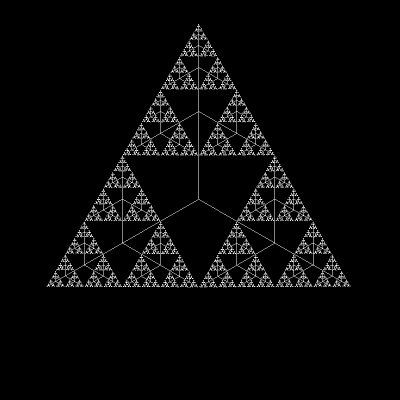Or how about some 3D fractals: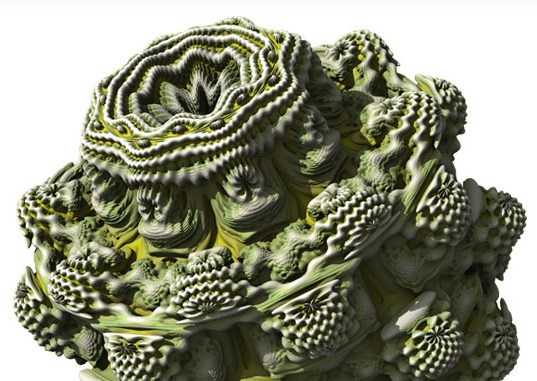You can even grow broccoli with fractals (yes this is real broccoli that you can buy at some supermarkets):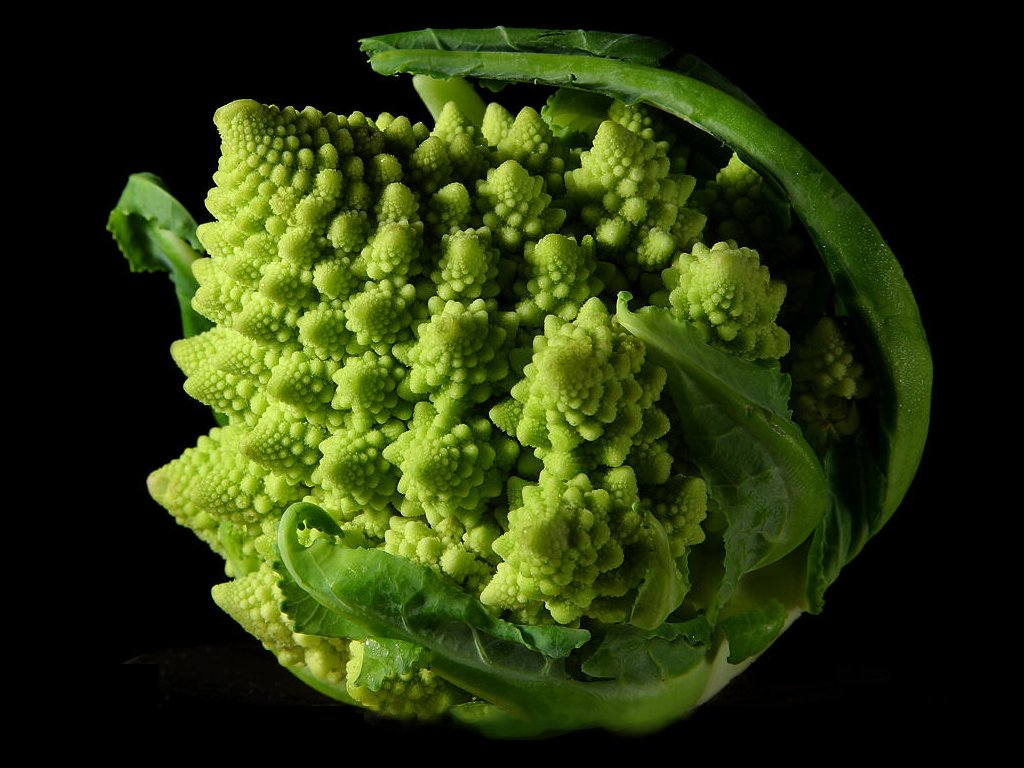But fractals are just the beginning of what you can do with complex numbers. Complex numbers are essential to everything from quantum physics to guitar amplifiers.

Pretty remarkable for such a simple idea.

Want to learn more stuff like this? I recommend checking out 3Blue1Brown.

### Sources

You are viewing a single comment. View All
RojerGS (9)

Great tutorial! Very well detailed! And it's funny you mention fractals in broccoli, I just wrote about them some days ago!! You can check it here if you want.